Command Reference : Object and Command Basics : Object Declaration and Initialization

Object Declaration and Initialization
The simplest types of commands create an EViews object, or assign data to or initialize an existing object.
Object Declaration
A simple object declaration has the form
object_type(options) object_name
where object_name is the name you would like to give to the newly created object and object_type is one of the following object types: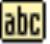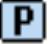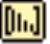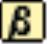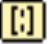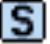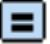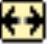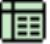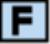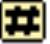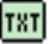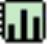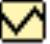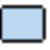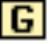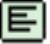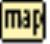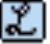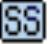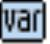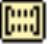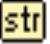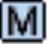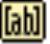For example, the declaration,
series lgdp
creates a new series called LGDP, while the command:
equation eq1
creates a new equation object called EQ1.
Matrix objects are typically declared with their dimension as an option provided in parentheses after the object type. For example:
matrix(5,5) x
creates a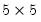matrix named X, while
coef(10) results
creates a 10 element coefficient vector named RESULTS.
Simple declarations initialize the object with default values; in some cases, the defaults have a meaningful interpretation, while in other cases, the object will simply be left in an incomplete state. In our examples, the newly created LGDP will contain all NA values and X and RESULTS will be initialized to 0, while EQ1 will be simply be an uninitialized equation containing no estimates.
Note that in order to declare an object you must have a workfile currently open in EViews. You may open or create a workfile interactively from the File Menu or drag-and-dropping a file onto EViews (see “Workfile Basics” for details), or you can may use the wfopen command to perform the same operations inside a program.
Object Assignment
Object assignment statements are commands which assign data to an EViews object using the “=” sign. Object assignment statements have the syntax:
object_name = expression
where object_name identifies the object whose data is to be modified and expression is an expression which evaluates to an object of an appropriate type. Note that not all objects permit object assignment; for example, you may not perform assignment to an equation object. (You may, however, initialize the equation using a command method.)
The nature of the assignment varies depending on what type of object is on the left hand side of the equal sign. To take a simple example, consider the assignment statement:
x = 5 * log(y) + z
where X, Y and Z are series. This assignment statement will take the log of each element of Y, multiply each value by 5, add the corresponding element of Z, and, finally, assign the result into the appropriate element of X.
Similarly, if M1, M2, and M3 are matrices, we may use the assignment statement:
m1 = @inverse(m2) * m3
to postmultiply the matrix inverse of M2 by M3 and assign the result to M1. This statement presumes that M2 and M3 are suitably conformable.
Object Modification
In cases where direct assignment using the “=” operator is not allowed, one may initialize the object using one or more object commands. We will discuss object commands in greater detail in a moment (see “Object Commands”) but for now simply note that object commands may be used to modify the contents of an existing object.
For example:
eq1.ls log(cons) c x1 x2
uses an object command to estimate the linear regression of the LOG(CONS) on a constant, X1, and X2, and places the results in the equation object EQ1.
sys1.append y=c(1)+c(2)*x
sys1.append z=c(3)+c(4)*x
sys1.ls
adds two lines to the system specification, then estimates the specification using system least squares.
Similarly:
group01.add gdp cons inv g x
adds the series GDP, CONS, INV, G, and X to the group object GROUP01.
More on Object Declaration
Object declaration may often be combined with assignment or command initialization. For example:
series lgdp = log(gdp)
creates a new series called LGDP and initializes its elements with the log of the series GDP. Similarly:
equation eq1.ls y c x1 x2
creates a new equation object called EQ1 and initializes it with the results from regressing the series Y against a constant term, the series X1 and the series X2.
Lastly:
group group01 gdp cons inv g x
create the group GROUP01 containing the series GDP, CONS, INV, G, and X.
An object may be declared multiple times so long as it is always declared to be of the same type. The first declaration will create the object, subsequent declarations will have no effect unless the subsequent declaration also specifies how the object is to be initialized. For example:
smpl @first 1979
series dummy = 1
smpl 1980 @last
series dummy=0
creates a series named DUMMY that has the value 1 prior to 1980 and the value 0 thereafter.
Redeclaration of an object to a different type is not allowed and will generate an error.